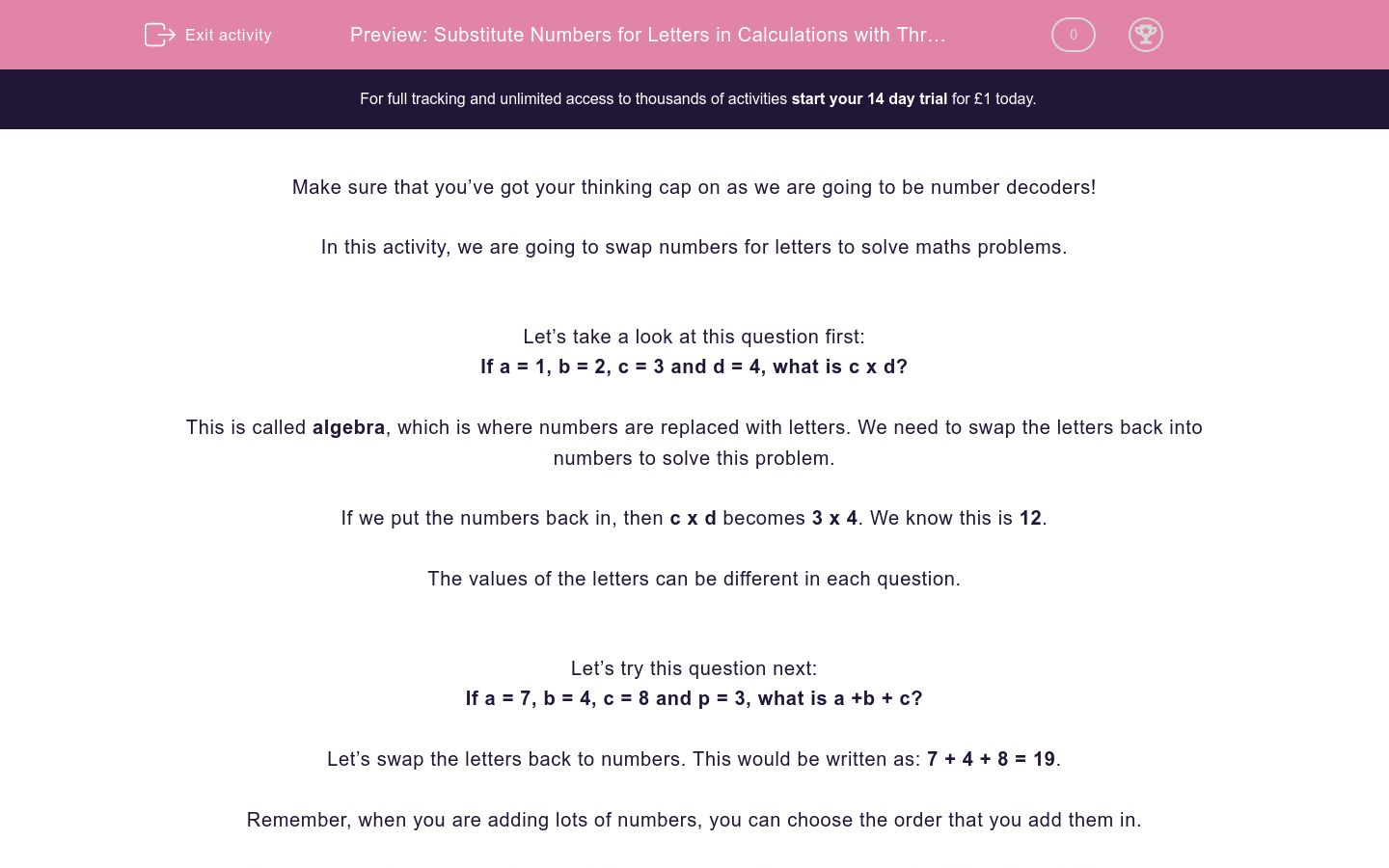# Substitute Numbers for Letters in Calculations with Three Steps

In this worksheet, students will replace letters with numbers and carry out simple questions using the four number operations. (The student will type the answer as a number and no prioritisation of calculations is required at this stage.)Key stage:  KS 2

Curriculum topic:   Verbal Reasoning

Curriculum subtopic:   Maths Codes

Difficulty level:#### Worksheet Overview

Make sure that you’ve got your thinking cap on as we are going to be number decoders!

In this activity, we are going to swap numbers for letters to solve maths problems.

Let’s take a look at this question first:

If a = 1, b = 2, c = 3 and d = 4, what is c x d?

This is called algebra, which is where numbers are replaced with letters. We need to swap the letters back into numbers to solve this problem.

If we put the numbers back in, then c x d becomes 3 x 4. We know this is 12.

The values of the letters can be different in each question.

Let’s try this question next:

If a = 7, b = 4, c = 8 and p = 3, what is a +b + c?

Let’s swap the letters back to numbers. This would be written as: 7 + 4 + 8 = 19.

Remember, when you are adding lots of numbers, you can choose the order that you add them in.

If there is a mixture of addition and subtraction, you must work it out in the order that it is written.

Let’s practise this now:

If a = 10, b = 4, c = 3 and d = 9, what is b + d - a + c?

Let’s swap the letters back to numbers. This would be written as: 4 + 9 - 10 + 3 =

Remember, we must answer this one in order.

So 4 + 9 = 13…

13 - 10 = 3…

3 + 3 = 6.

Now it’s your turn to crack the codes. Good luck number detective!

### What is EdPlace?

We're your National Curriculum aligned online education content provider helping each child succeed in English, maths and science from year 1 to GCSE. With an EdPlace account you’ll be able to track and measure progress, helping each child achieve their best. We build confidence and attainment by personalising each child’s learning at a level that suits them.

Get started